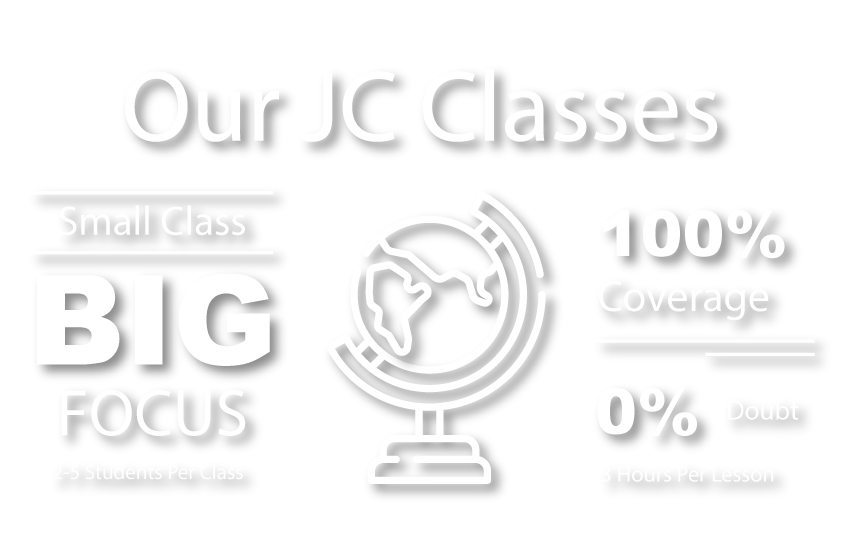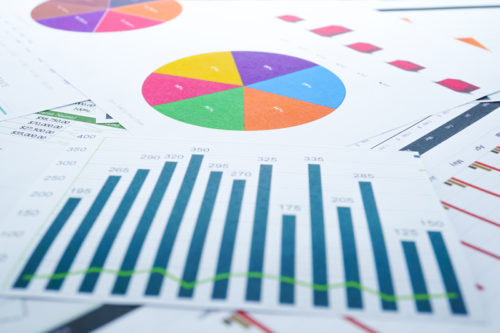# JC H2 Math Tuition

## JC1 TO JC2 A-LEVEL H2 MATHEMATICS

### Pure Mathematics

At Einstein Education Hub, we have a strong specialization in JC H2 Math. Our JC H2 Math tuition classes are tailored to suit the learning needs of our JC students. Need a good H2 math tutor in Singapore to guide your child? Our H2 math tutors are experienced and our lesson plans are all up to date with the latest JC H2 Math syllabus and examinations.## Our Achievements# 14

YEARS OF EXPERTISE
IN EDUCATION# 100%

OF OUR JC STUDENTS FIND OUR LESSONS SUPER CLEAR AND COMPREHENSIVE# 5

STUDENTS MAXIMUM IN A CLASS. FULL ATTENTION AND GUIDANCE##### Functions and graphs
• Use graphing calculator (GC) to sketch various functions and label necessary points.
• Determine its domain and range from the graph.
• Determine the equations of asymptotes using algebraic methods.
• Use proper notations in functions and graphs.
• Find inverse functions, composite functions and state their necessary conditions.
• Establish graphical relationship between a function and its inverse.
• Sketch the effects of transformations on graph by identifying the correct sequence of transformation.
• Formulate a system of linear equations or inequalities from the situation given (for example optimisation problems) and solve them graphically and algebraically.

##### Sequence and series
• Master the concepts of sequence and series for finite and infinite cases and its conditions for convergence with the use of appropriate notation.
• Form suitable equation for the nth term and sum of terms given a sequence or pattern by using formula in arithmetic progression, geometric progression or method of difference.
• Relate the concept to real life situation such as financial maths

##### Vectors
• Comprehend important terms used in vectors such as position vectors, unit vectors and length of projection.
• Calculate and interpret geometrically vectors’ magnitude, direction, dot product, cross product, angle, length of projection, perpendicular distance and foot of perpendicular.
• Use ratio theorem in geometrical application questions.
• Interpret given information to form vector, cartesian and parametric equations of lines and planes.
• Describe geometrical and algebraic relationships between lines and planes.
##### Complex numbers
• Interchange of cartesian form and polar form of complex number.
• Solve complex roots of quadratic equations with real coefficients by making use of conjugate roots properties.
• Basic operations of complex numbers and solving using algebraic method.
• Represent complex numbers in the Argand diagram.
• Link the concepts to electrical circuits.

##### Calculus
• Sketch y = f′(x) from y = f(x) or vice versa.
• Differentiate functions defined implicitly or parametrically.
• Findstationary points and their nature algebraically and graphically.
• Find equations of tangents and normals to curves.
• Solve rates of change problems given real-life problems by using chain rule.
• Manipulate the standard series expansionformula given in MF26to solve questions related to Maclaurin series and state the range of values of x for which it converges.
• Apply differentiation repeatedly to find the terms in Maclaurin expansion.
• Substitute appropriate value of x in order to use Maclaurin series for approximation.
• Integrate functions using formulae, substitution and by parts methods.
• Identify the appropriate functions and limits when using definite integral to find area bounded by curves and volume of revolution about the x-axis or y-axis.
• Master the use of GC wherever possible to find an approximate value of a definite integral and differentiation of function at given point.
• Form differential equations from a real-life scenario such as population growth, radioactive decay, heating and cooling problems.
• Solve general solutions and particular solutions of differential equations using integration.
• Explain the importance of the solution based on the context of the question.

### Statistics##### Permutation, combination and probability
• Use concepts of permutation and combination to find the arrangements of objects in a line or in a circle, including cases involving repetition and restriction.
• Comprehend the terms mutually exclusive events and independent events.
• Use tables of outcomes, Venn diagrams, tree diagrams, permutations and combinations techniques to calculate probabilities including conditional probabilities.

##### Discrete and continuous distribution
• Comprehend the concept of discrete random variable, tabulate its probability distribution and calculate its expectation and variance.
• Find the mean and variance of binomial distributionand represent it as B(n, p).
• State the necessary conditions where binomial distribution is a suitable model.
• Represent normal distribution as an example of a continuous probability model with mean, µ and variance, σ2.
• Standardise normal distribution and use the symmetry of the normal curve and its properties to find its unknown parameters
• Solve problems involving the use of the distribution aX + bY + c where X and Y are independent.
• Know the differences between population, random and non-random samples, sample mean and sample variance.
• Use Central Limit Theorem to treat sample means as having normal distribution when the sample size is sufficiently large.
• Calculate unbiased estimates of the population mean and variance from a sample.
• Master the use of GC to find the required probability and parameters for various distribution.

##### Hypothesis testing
• Comprehend the concepts of null hypothesis, alternative hypotheses, test statistic, critical region, critical value, level of significance and p-value.
• Write the proper steps in conducting hypothesis testing.
• Interpretthe results of a hypothesis test in the context of the problem such as standardised testing, market research and clinical research.
##### Correlation and Linear regression
• With the help of GC, use scatter diagram and product moment correlation coefficient to determine if there is alinear relationship between two variables.
• Calculate the product moment correlation coefficient using its formula and discuss its significance in the context of the question.
• Use GC to find theequation of the regression line using the given information.
• Estimate the values of x or y in practical situations using the appropriate regression line.
• Determine if interpolation and extrapolation can be applied to approximate given values in the situation given.
• Transform given graph to achieve linearity.

### Application Questions• To be exposed on different types of problem-solving questions and link it to other related disciplines like sciences and engineering.
• Develop proper thinking and reasoning skills to solve mathematical problems.
• Identify and apply the relevant mathematical concepts and skills in various problems, including unfamiliar contexts.
• Link concepts from different topics together to solve given problems.
• Apply the concepts correctly by having a clear thought process and showing all necessary steps.
• Master the use of GC and to solve the questions more efficiently.
• Solve the mathematical problems and explain its significance in the context of the problems.